# Summing Values from Two Ranges, Based on Two Criteria

To sum the values from two ranges based on two criteria, we will use SUMIFS function and SUMPRODUCT function in Microsoft Excel.

SUMIFS: This function is used for adding the cells specified by a given set of condition or criteria.

The syntax of SUMIFS formula:-  =SUMIFS(sum_range,criteria_range1,criteria1,…)

Let’s take an example to understand how we can use the SUMIFS function.

We have sales data in range A1:C15. Column A contains agent's name, column B contains City name, and column C contains sales amount.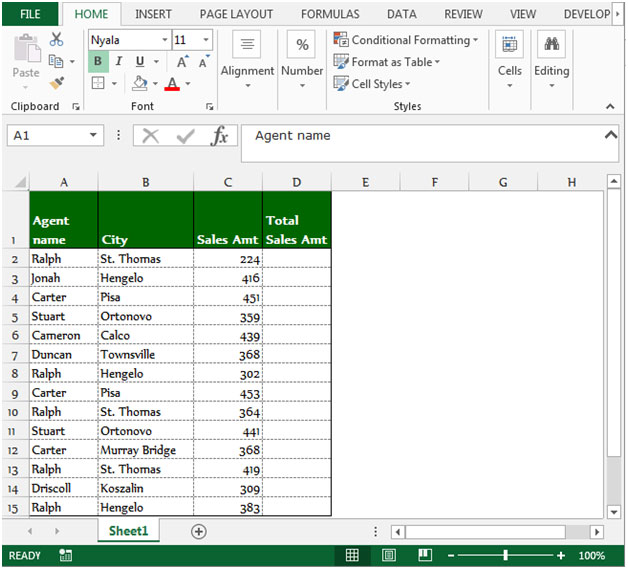In the above shown picture, we have data in Excel like this. We want to return the total value of every agent and agent should be from the same city.

To return the total value agent wise, follow below given steps:-

• Select the cell D2.
• Write the formula.
• =SUMIFS(C:C,A:A,A2,B:B,B2), press Enter on your keyboard.
• The function will return the total value for the agent Ralph and his city is St. Thomas.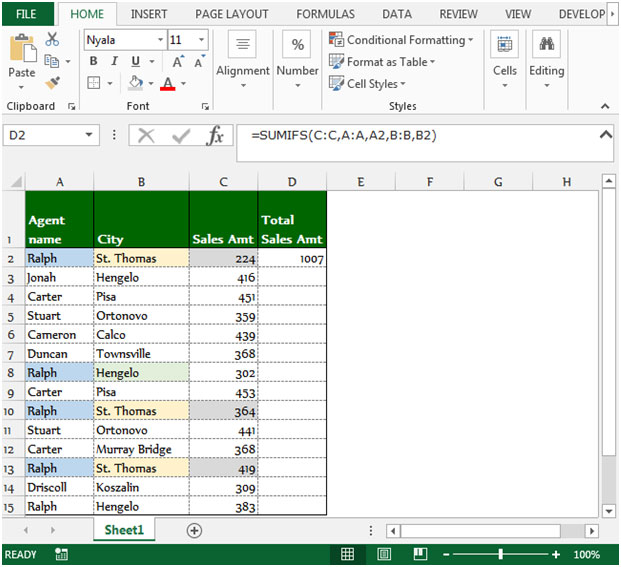To return the total amount for all the agents, copy the same formula by pressing the key Ctrl+C and paste in the range D3:D15 by pressing the key Ctrl+V on your keyboard.

Sum product in Excel is a multipurpose function which will provide different results depending on the way the function’s arguments are entered. The purpose of “Sum product” is to multiply two or more ranges and then sum them in Microsoft Excel 2010 and 2013.

Syntax of “SUMPRODUCT” function:

=sum product (array1, array2, array3……….).

Let’s understand with a simple exercise how we can use the Sumproduct function with multiple criteria.

We have a table in Range “A2 to C16”. We need to calculate the total score of Agent 4 in cell C18. Let’s see how we can use the sumproduct function to return this value.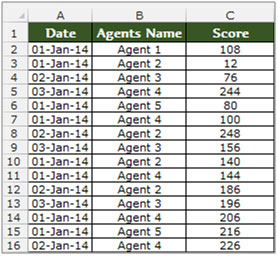• Select the cell C18, and write the formula in the cell.
• =SUMPRODUCT((B2:B16=B18)*(C2:C16))
• Press Enter on the keyboard.
• The function will return the score value of Agent 4.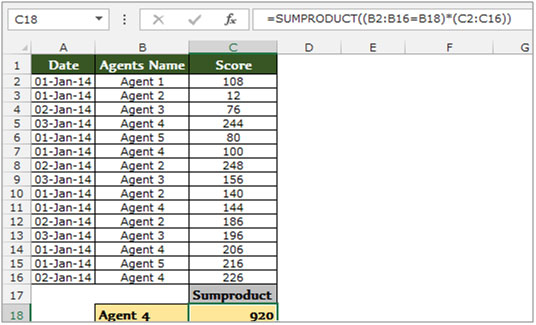Let’s take an example to understand how we can sum values from two ranges based on two criteria.

We have a table in Range “A2 to C10” which contains the Agents score data. Column A contains date, Column B Agents Name and Column C contains score. Now we need to calculate the total score of Agent 4 in cell C18. Let’s see how we can use the sumproduct function to return this value.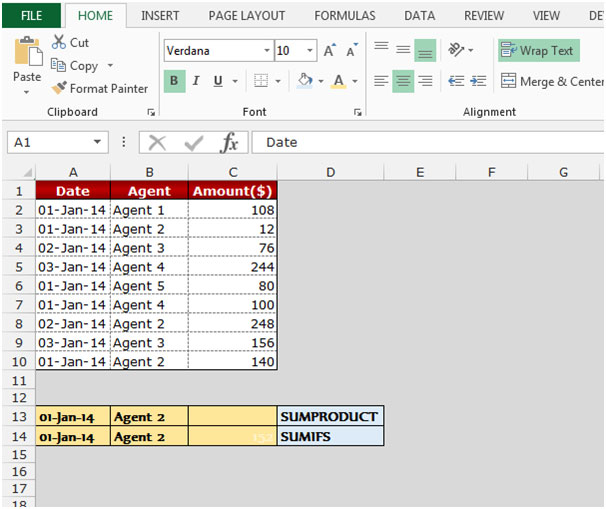Using SUMPRODUCT function:-

If we require the total score of Agent 2 only for 1st Jan’2014, then we need to follow below given steps: -

• Select the cell C13, and write the formula in the cell.
• =SUMPRODUCT((B2:B10=B13)*(A2:A10=A13)*(C2:C10))
• Press Enter on the keyboard.
• The function will return the score value of Agent 2 as on 1st-Jan-2014.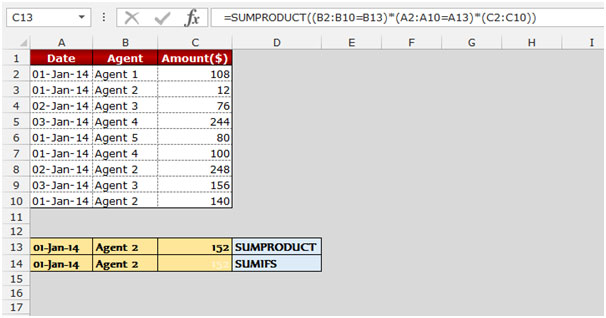Using SUMIFS function:-

• Select the cell C14, and write the formula in the cell.
• =SUMIFS(C2:C10,A2:A10,A14,B2:B10,B14)
• Press Enter on the keyboard.
• The function will return the score value of Agent 2 as on 1st-Jan-2014.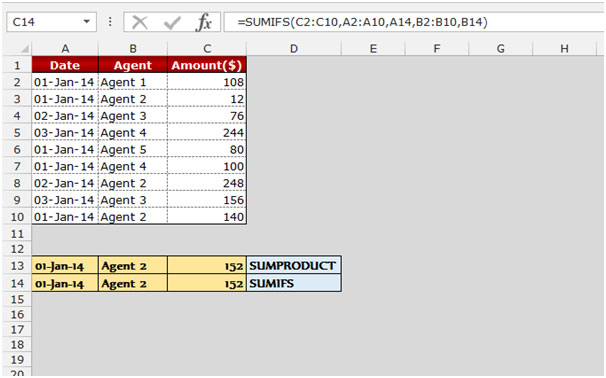These are the ways we can return the total from two ranges, based on two criteria in Microsoft Excel.

Terms and Conditions of use

The applications/code on this site are distributed as is and without warranties or liability. In no event shall the owner of the copyrights, or the authors of the applications/code be liable for any loss of profit, any problems or any damage resulting from the use or evaluation of the applications/code.